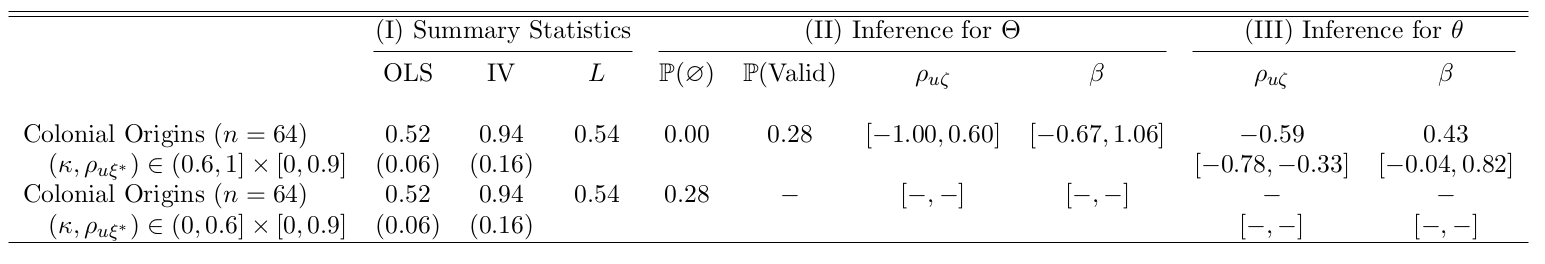# Example 1: Colonial Origins

This vignette illustrates how to use the ivdoctr package in practice. This example comes from “The colonial origins of comparative development: An empirical investigation” by Acemoglu, Johnson, and Robinson (2001).

## Empirical Model

The authors study the effect of institutions on GDP per capita across 64 countries. Since institutional quality is endogenous, they use differences in mortality rates of early western settlers across colonies as an instrumental variable. The regression specification is as follows:

\begin{align*} \log(GDP / capita) &= \alpha_0 + \beta Institutions + u \\ Institutions &= \alpha_1 + \pi \log(Settler Mortality) + v \end{align*}

The authors state that there is likely a positive correlation between institutional quality and the error term ($$u$$), which could come from reverse causality (e.g., wealthier societies can afford better institutions) or omitted variables (e.g., rule of law or British culture are positively correlated with present-day institutional quality). This positive correlation is a researcher belief that can be input into ivdoctr using the r_TstarU_restriction argument that accepts a 2-element vector of bounds. For the exercise, we use 0.9 as the conservative upper bound on the extent of the endogeneity.

The authors also state that up to 40% of the measure of “institutions” is noise. Measurement error is $$1-\kappa$$, so $$\kappa \in [0.6, 1]$$ is the translation of this belief. The code below runs the estimation given these beliefs:

library(ivdoctr)
endog <- c(0, 0.9)
meas <- c(0.6, 1)

colonial_example1 <- ivdoctr(y_name = "logpgp95", T_name = "avexpr",
z_name = "logem4", data = colonial,
controls = NULL, robust = FALSE,
r_TstarU_restriction = endog,
k_restriction = meas,
example_name = "Colonial Origins")

Let’s say the reader wants to examine how these results might change if the measurement error is worse than the researchers allowed for. The following code implements the worst case scenario, allowing for all information to be noise (we need to be just a little away from 0 to prevent computation errors).

endog <- c(0, 0.9)
meas <- c(0.001, 0.6)

colonial_example2 <- ivdoctr(y_name = "logpgp95", T_name = "avexpr",
z_name = "logem4", data = colonial,
controls = NULL, robust = FALSE,
r_TstarU_restriction = endog,
k_restriction = meas,
example_name = "Colonial Origins")

Now, we can generate the LaTeX summary table illustrating these estimation results using makeTable() and save it to colonial.tex.

makeTable(colonial_example1, colonial_example2, output = "colonial.tex")

This generates the following table:To explore the surface of estimates consistent with Autor et al.’s beliefs, ivdoctr also generates an interactive 3D plot of the surface, which can be rotated and zoomed using the mouse:

library(ivdoctr)
endog <- c(0, 0.9)
meas <- c(0.6, 1)

plot_3d_beta(y_name = "logpgp95", T_name = "avexpr", z_name = "logem4",
data = colonial, r_TstarU_restriction = endog, k_restriction = meas)

## Usage

This package exports three main functions:

• ivdoctr(): Generates list of estimates, including OLS and IV regression objects
• makeTable(): Generates the TeX code for a stand-alone regression table and saves it to the specified file.
• plot_3d_beta(): Generates an interactive 3D plot illustrating the relationship between the causal estimates, instrument endogeneity, instrument invalidity, and measurement error.

Both ivdoctr and plot_3d_beta use the same primary inputs. Users input the name of the dataset (data), the name of the dependent variable (y_name), the name of the treatment variable(s) (T_name), the name(s) of the instrument(s) (z_name), and the names of the control variables (controls). Without any additional arguments, the functions will output the identified set. If users have beliefs over measurement error and/or instrument endogeneity, they can specify those using k_restriction and r_TstarU_restriction, respectively.